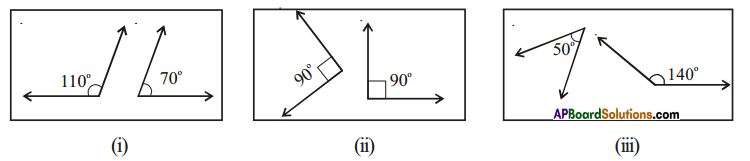# AP Board 7th Class Maths Solutions Chapter 4 Lines and Angles Ex 3

AP State Syllabus AP Board 7th Class Maths Solutions Chapter 4 Lines and Angles Ex 3 Textbook Questions and Answers.

## AP State Syllabus 7th Class Maths Solutions 4th Lesson Lines and Angles Exercise 3Question 1.
Which of the following pairs of angles are supplementary?Solution:
i) Sum of the angles = 110° + 70° =180°
Hence they are supplementary.

ii) Sum of the angles = 90° + 90° =180°
hence they are supplementary.

iii) Sum of the angles = 50°+ 140° =190° ≠ 180°
Hence they are not supplementary.Question 2.
Find the supplementary angles of the given angles.
(i) 105° (ii) 95° (iii) 150° (iv) 20°
Solution:
(i) 105°
Supplementary angle of 105° = 180° – 105° = 75°
(ii) 95°
Supplementary angle of 95° = 180° – 95° = 85
(iii) 150°
Supplementary angle of 150° = 180° – 150° = 30°
(iv) 20°
Supplementary angle of 20° = 20° = 180° – 20° = 60°

Question 3.
Two acute angles cannot form a pair of supplementary angles. Justify.
Solution:
As the measure of an acute angle is less than 90°, sum of two acute angles < 90° + 90° = 180°
Hence two acute angles cant brin a pair of supplementary angles.Question 4.
Two angles are equal and supplementary to each other. Find them.
Solution:
Let the angle be x°
then its supplementary 180° – x°
By problem, x° =180° – x°
x° + x° = 180°
x° = $$\frac{180^{\circ}}{2}$$
x° = 90°